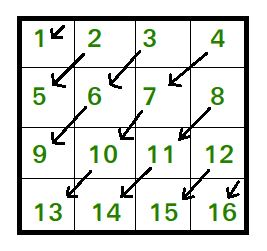# Print the matrix diagonally downwards

Given a matrix of size n*n, print the matrix in the following pattern.Output: 1 2 5 3 6 9 4 7 10 13 8 11 14 12 15 16

Examples:

```Input :matrix= { {1, 2},
{3, 4} }
Output : 1 2 3 4

Input :matrix= { {1, 2, 3},
{4, 5, 6},
{7, 8, 9} }
Output : 1 2 4 3 5 7 6 8 9
```

## Recommended: Please try your approach on {IDE} first, before moving on to the solution.

Following is the C++ implementation for the above pattern.

## C++

 `// CPP program to print matrix downward ` `#include ` `using` `namespace` `std; ` ` `  `void` `printMatrixDiagonallyDown(vector > matrix,  ` `                                                    ``int` `n) ` `{ ` `    ``// printing elements above and on  ` `    ``// second diagonal ` `    ``for` `(``int` `k = 0; k < n; k++) { ` ` `  `        ``// traversing downwards starting ` `        ``// from first row ` `        ``int` `row = 0, col = k; ` `        ``while` `(col >= 0) { ` `            ``cout << matrix[row][col] << ``" "``; ` `            ``row++, col--; ` `        ``} ` `    ``} ` ` `  `    ``// printing elements below second ` `    ``// diagonal ` `    ``for` `(``int` `j = 1; j < n; j++) { ` ` `  `        ``// traversing downwards starting  ` `        ``// from last column ` `        ``int` `col = n - 1, row = j; ` `        ``while` `(row < n) { ` `            ``cout << matrix[row][col] << ``" "``; ` `            ``row++, col--; ` `        ``} ` `    ``} ` `} ` ` `  `int` `main() ` `{ ` `    ``vector > matrix{ { 1, 2, 3 }, ` `                                 ``{ 4, 5, 6 }, ` `                                 ``{ 7, 8, 9 } }; ` `    ``int` `n = 3; ` `    ``printMatrixDiagonallyDown(matrix, n); ` `    ``return` `0; ` `} `

## Python 3

 `# Python 3 program to print matrix downward ` ` `  `def` `printMatrixDiagonallyDown(matrix,n): ` `    ``# printing elements above and on  ` `    ``# second diagonal ` `    ``for` `k ``in` `range``(n): ` `        ``# traversing downwards starting ` `        ``# from first row ` `        ``row ``=` `0` `        ``col ``=` `k ` `        ``while` `(col >``=` `0``): ` `            ``print``(matrix[row][col],end ``=` `" "``) ` `            ``row ``+``=` `1` `            ``col ``-``=` `1` ` `  `    ``# printing elements below second ` `    ``# diagonal ` `    ``for` `j ``in` `range``(``1``,n): ` `        ``# traversing downwards starting  ` `        ``# from last column ` `        ``col ``=` `n ``-` `1` `        ``row ``=` `j ` `        ``while` `(row < n): ` `            ``print``(matrix[row][col],end ``=` `" "``) ` `            ``row ``+``=` `1` `            ``col ``-``=` `1` ` `  `if` `__name__ ``=``=` `'__main__'``: ` `    ``matrix ``=` `[[``1``, ``2``, ``3``],[``4``, ``5``, ``6``],[``7``, ``8``, ``9``]] ` `    ``n ``=` `3` `    ``printMatrixDiagonallyDown(matrix, n) ` ` `  `# This code is contributed by Surendra_Gangwar `

Output:

```1 2 4 3 5 7 6 8 9
```

Time Complexity: O(n*n)

Attention reader! Don’t stop learning now. Get hold of all the important DSA concepts with the DSA Self Paced Course at a student-friendly price and become industry ready.

My Personal Notes arrow_drop_upCheck out this Author's contributed articles.

If you like GeeksforGeeks and would like to contribute, you can also write an article using contribute.geeksforgeeks.org or mail your article to contribute@geeksforgeeks.org. See your article appearing on the GeeksforGeeks main page and help other Geeks.

Please Improve this article if you find anything incorrect by clicking on the "Improve Article" button below.